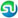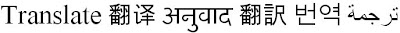Give it a thumb# Introduction to Quantum Theory

Quantum Mechanics has been described as one of the crowning achievements of the 20th Century, a theory that has been validated to a precision of one part in 10 8. The central concept of this new theory is that of the quanta - the idea that at the smallest of scales everything is discrete. But, from where did such a dramtic concept arise?

## Ultra-violet CatastropheDuring the 19th century much attention was given to the study of heat properties of various objects. An idealised model that was considered was the Black Body, an object which absorbs all incident radiation and then re-emits all this energy again. We can think of the radiating energy as standing waves inside our blackbody cavity. The energy of the radiating waves at a given frequency ˝, should be proportional to the number of modes at this frequency. Classical physics states that all these modes have the same energy k T (a result derived from classical thermodynamics) and as the number of modes is proportional to ˝ 2 :This implies that we would expect most of the energy at higher frequency, and this energy diverges with frequency. If we try and sum the energies at each frequency we find that there is an infinite energy in ths system! This paradox was called the ULTRAVIOLET CATASTROPHE.Max Planck

It was left to Planck to resolve this gaping paradox. He postulated that the energy of the modes could only come in discrete packets - quanta - of energy:Using statistical mechanics Planck found that the modes at higher frequency were less likely excited so the average energy of these modes would decrease with the frequency. The exact expression for the average energy of each mode is given by the Planck distribution:You can see that if the frequency is low then the average energy tends towards the classical result, and as frequency goes to infinity we get that the average energy goes to zero as expected.

## Atomic Line SpectraThere was a further breakdown in classical physics to the observed discrete line spectra specific to each atom. It was widely accepted that this was due to transitions between atomic energy levels. This presented a considerable problem to classical physics as it predicted that accelerating charges emitted radiation. It would thus seem that the energy orbital would be unstable as the electron would slowly spiralled towards the nuclei as it emitted radiation. There was no classical model which could explain stable electron orbitals.Niels Bohr

Bohr was able to crack this puzzle by invoking the idea developed by Planck. He postualted that the angular momentum, given by mvr, was quantised in units of h. He realised that if use this single constraint then we obtain the following relation which describes the energy of the orbital levels.

[you can play around with the Bohr model of the atom here]:This magical conjuring act was able to accurately describe the energy levels giving the exact energies of the lines observed in the atomic spectra.

Although this gave the exact energies required, a better explanation for these energy levels required further work, and the use of Schrodingers Equation, of which we will learn later on.

# Heisenberg's Uncertainty PrincipleQuantum mechanics is a probabilistic theory, and it is this randomness that places limitations on the accuracy of characterizing a system.

Let us consider a particle, say an electron, moving through space. We describe the electron's motion in terms of its position and momentum. Classically we can measure both quantities to infinite precision. However in Quantum Mechanics we can never know both quantities absolutely precisely. This is because by taking a measurement we inadvertantly disturb the system as well.Werner Heisenberg

Heisenberg was able to quantify this effect through his Uncertainty Principle (HUP) which states that measured values cannot be applied to both the position x and momentum p of a particle with unlimited precision but instead they follow the relation:Hence ” x and ” p represent intrinsic uncertainies in the measurement of x and p.

Unfortunately this is something that we just cannot avaid. We can only deal in probabilities, so all we can say is that the probability that the electron is here is say 75%.

A good example of this is, if we consider a free particle with a definite momentum, sayp = 0, then its position is given by the sine function, and so the particle can be anywhere in space, i.e.. We can try localising it in space by adding a number of similar sine waves with slightly different momenta. Then we get the following probability distributions for the position and momentum:

# Wave and Particle Duality

## What is the nature of light? Wave ... or particle?## Young's Double Slit experiment: Light behaves like a wave

If we point a light source ( e.g. laser pointer) at a double slit, we observe an interference pattern similar to what we would observe for water waves.

## Water waves & interference:

If we tap water in a tank in the centre we see concentric circles of crests and troughs travelling outward from the point of agitation. If this wave hits a barrier with two holes in it, two new waves form.When they meet we observe that in some places the crests of each wave coincide and amplify. This is called constructive interference. On other points, the crests of one wave and the troughs of the other wave coincide and cancel each other. This is called distructive interference. The resulting pattern is an interference pattern.If light was a stream of particles, such as bullets from a gun, we would expect a much simpler pattern. The distribution of the bullet would simply be the sum (blue) of the distributions of bullets going through slit 1 (red) and 2 (green), respectively, as shown in the figure to the right.So Young's double slit experiment conclusively shows that light is a wave, or does it?

## The Photoelectic effect: Light behaves like a particleAlbert Einstein

When light is shone on some metals, it produces a small current. This is rather easy to explain: the light carries energy, and if there is enough of it, it may knock out some of the electrons present in the metal. It was indeed observed that the number of electrons knocked out increased with the brightness of the light source, BUT ONLY for light of certain colours (frequencies). Below a certain threshold frequency, no electrons were observed, no matter how bright the light source. This didn't make sense at all! If light was a wave then the energy of light should depend only on its brightness, not its frequency.Einstein's genius: If we assume that light is a stream of discrete parcels of energy (photons), and the energy of these photons is proportional to the colour of the light, we can easily explain the observed threshold  photons below a certain frequency don't have enough energy to knock out any electrons. This simple explanation earned Einstein the Nobel prize.

The photoelectric effect is exploited in many applications from solar cells to charge-coupled devices that record images in digital cameras.

## Dual behavior is a general phenomenon

The previous section has shown that light exhibits both wave-like and particle-like behaviour. But this strange behavior is not limited to light.Matter particles from electrons to fairly large objects such as Carbon-60 have been shown to exhibit both wave and particle-like behavior.

What, you may be asking, does all this have to do with yoga? I'll give you a big hint... When you read wave and particle, think Purusha and Prakriti.